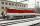# Reducing balance method

A company buys an item having a useful life of 10 years for 1,000,000. If the company depreciates the item by the reducing balance method,
a. Determine the depreciation for the first year.
b. Estimate the depreciation for the second and third years.
c. What is the salvage value of the asset?

Result

a =  100000
b2 =  90000
b3 =  81000
c =  348678.44

#### Solution:

$t = 10 \ y \ \\ x = 1000000 \ \\ \ \\ q = 1/t = 1/10 = \dfrac{ 1 }{ 10 } = 0.1 \ \\ a = x \cdot \ q = 1000000 \cdot \ 0.1 = 100000 = 1.000000\cdot 10^{ 5 }$
$b_{ 2 } = q \cdot \ (x - a) = 0.1 \cdot \ (1000000 - 100000) = 90000$
$b_{ 3 } = q \cdot \ (x - a-b_{ 2 }) = 0.1 \cdot \ (1000000 - 100000-90000) = 81000$
$k_{ 10 } = (1-q)^{10} = (1-0.1)^{10} \doteq 0.3487 \ \\ c = k_{ 10 } \cdot \ x = 0.3487 \cdot \ 1000000 = 348678.4401 = 348678.44$

Leave us a comment of this math problem and its solution (i.e. if it is still somewhat unclear...):Be the first to comment!## Next similar math problems:

1. Reconstruction of the corridorCalculate how many minutes will be reduced to travel 187 km long railway corridor, where the maximum speed increases from 120 km/h to 160 km/h. Calculate how many minutes will shorten travel time, if we consider that the train must stop at 6 stations, eac
2. CanisterGasoline is stored in a cuboid canister having dimensions 44.5 cm, 30 cm, 16 cm. What is the total weight of a full canister when one cubic meter of gasoline weighs 710 kg and the weight of empty canister is 1.5 kg?
3. Cu thiefThe thief stole 122 meters copper wire with cross-section area of 95 mm2. Calculate how much money gets in the scrap redemption, if redeemed copper for 5.5 eur/kg? The density of copper is 8.96 t/m3.
4. Tower modelTower height is 300 meters, weight 8000 tons. How high is the model of the tower weight 1 kg? (State the result in the centimeters). The model is made from exactly the same material as the original no numbers need to be rounded. The result is a three-digi
5. Copper plateCalculate the thickness of the copper plate with a density 8.7 g/cm³ measuring 1.5 meters and 80 cm and its weight is 3.65 kg
6. Traffic collisionWhen investigating a traffic accident, it was found that the driver stopped the vehicle immediately after the accident by constant braking on a 150 m track in 15 seconds. Do you approve that the driver exceeded the permitted speed (50 km/h) in the village.
7. A carA car weighing 1.05 tonnes driving at the maximum allowed speed in the village (50 km/h) hit a solid concrete bulkhead. Calculate height it would have to fall on the concrete surface to make the impact intensity the same as in the first case!
8. Orlík hydroelectric plantThe Orlík hydroelectric power plant, built in 1954-1961, consists of four Kaplan turbines. For each of them, the water with a flow rate of Q = 150 m3/s is supplied with a flow rate of h = 70.5 m at full power. a) What is the total installed power of the p
9. PumpWhat power has a pump output to move 4853 hl of water to a height of 31 m for 8 hours?
10. Copper sheetThe copper plate has a length of 1 m, width 94 cm and weighs 9 kg. What is the plate thickness, if 1 m3 weighs 8715 kg?
11. ClockHow many times a day hands on a clock overlap?
12. SphereThe surface of the sphere is 12100 cm2, and weight is 136 kg. What is its density?
13. Sphere slicesCalculate volume and surface of a sphere, if the radii of parallel cuts r1=31 cm, r2=92 cm and its distance v=25 cm.
14. Trains for peopleIt is said that the train is synonymous to delay. Calculate the average speed of travel by train long 85 km, with regular train leave at 7:00 and arrive at 8:18, but train is late and has departure at 8:10 and arrive at 9:27.
15. Cuboid 5Calculate the mass of the cuboid with dimensions of 12 cm; 0.8 dm and 100 mm made from spruce wood (density = 550 kg/m3).
16. Aluminum wireAluminum wire of 3 mm diameter has a total weight of 1909 kg and a density of 2700 kg/m3. How long is the wire bundle?
17. CarAt what horizontal distance reaches the car weight m = 753 kg speed v = 74 km/h when the car engine develops a tensile force F = 3061 N. (Neglect resistance of the environment.)